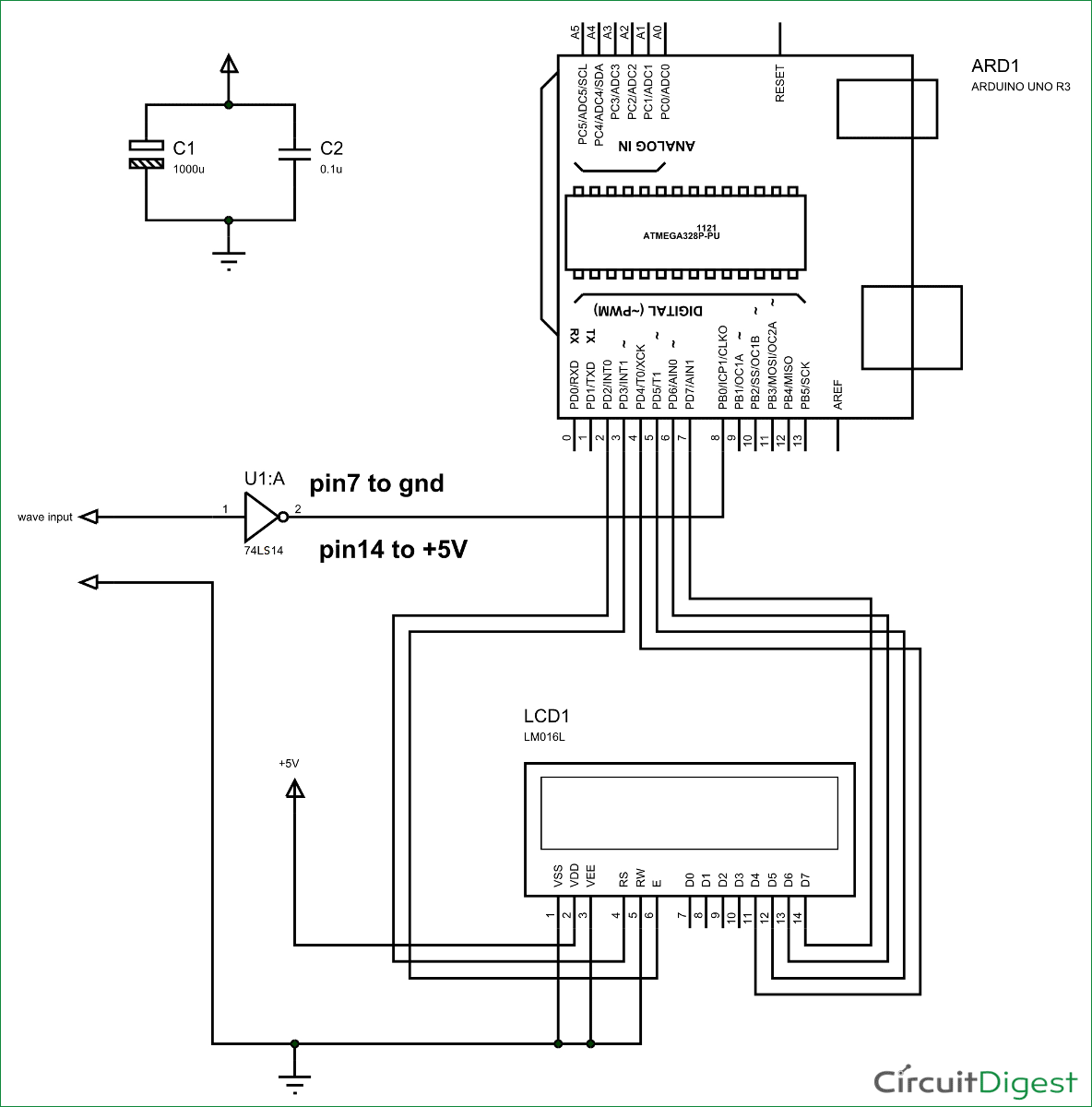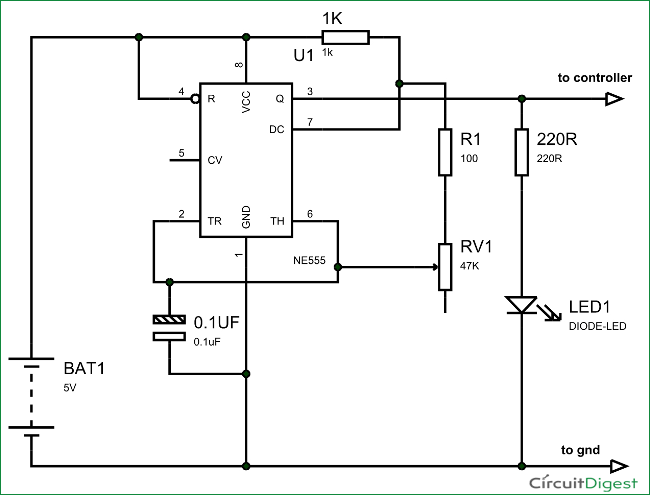# Frequency Counter using Arduino

Almost every electronic hobbyist must have faced a scenario where he or she must measure the frequency of signal generated by a clock or a counter or a timer.  We can use oscilloscope to do the job, but not all of us can afford an oscilloscope. We can buy equipment for measuring the frequency but all these devices are costly and are not for everyone. With that in mind we are going to design a simple yet efficient Frequency Counter using Arduino Uno and Schmitt trigger gate.This Frequency Counter is cost effective and can be easily made, we are going to use ARDUINO UNO for the measuring the frequency of signal, UNO is the heart of project here.

To test the Frequency Meter, we are going to make a dummy signal generator. This dummy signal generator will be made by using a 555 timer chip. The timer circuit generates a square wave which will be provided to UNO for testing.

With everything in place we will have a Frequency meter and a square wave generator.

### Required Components:

• 555 timer IC and 74LS14 Schmitt trigger gate or NOT gate.
• 1K Ω resistor(2 pieces), 100Ω resistor
• 100nF capacitor (2 pieces), 1000µF capacitor
• 16*2 LCD,
• 47KΩ pot,

### Circuit Explanation:

The circuit diagram of the Frequency Meter using Arduino is shown in below figure. Circuit is simple, a LCD is interfaced with Arduino to display the measured frequency of signal. ‘Wave Input’ is going to Signal Generator Circuit, from which we are feeding signal to Arduino. A Schmitt trigger gate (IC 74LS14) is used to ensure that only rectangular wave is fed to Arduino. For filtering the noise we have added couple of capacitors across power. This Frequency Meter can measure frequencies up to 1 MHz.Signal generator circuit and Schmitt trigger have been explained below.

###First of all we will talk about 555 IC based square wave generator, or should I say 555 Astable Multivibrator.  This circuit is necessary because, with the Frequency Meter in place we must have a signal whose frequency is known to us. Without that signal we will never be able to tell the working of Frequency Meter. If we have a square have of known frequency we can use that signal to test the UNO meter and we can tweak it for adjustments for accuracy, in case of any deviations. The picture of Signal Generator using 555 Timer IC is given below:

Read More:  Frequency Counter using Arduino

#### This Post / Project can also be found using search terms:

• For filtering the noise we have added couple of capacitors across power This Frequency Meter can measure frequencies up to 1 MHz Signal generator circuit and Schmitt trigger have been explained below
• fréquence meter via Arduino